• 文章指出，基于地理的时序预测，存在两个复杂的因素： 动态时空关联性 传感器之间的相关性，即空间相关性 传感器自身的相关性，即与历史时间的相关性 外部因素 传感器的读数会受到许多外部因素影响，像天气、...


文章目录
MSSTN: Multi-Scale Spatial Temporal Network for Air Pollution Prediction问题提出网络结构S-NetT-NetF-Net源码
数据集分析
GeoMAN: Multi-level Attention Networks for Geo-sensory Time Series Prediction问题提出网络结构空间注意力编码器时间注意力外部因素融合解码器

MSSTN: Multi-Scale Spatial Temporal Network for Air Pollution Prediction
问题提出
文章指出，对于空气质量数据集，无论是在时间维度和空间维度上，都存在着多尺度特性，例如，在时间方面存在短期波动，中期周期性和长期趋势，在空间方面存在城市规模和区域规模。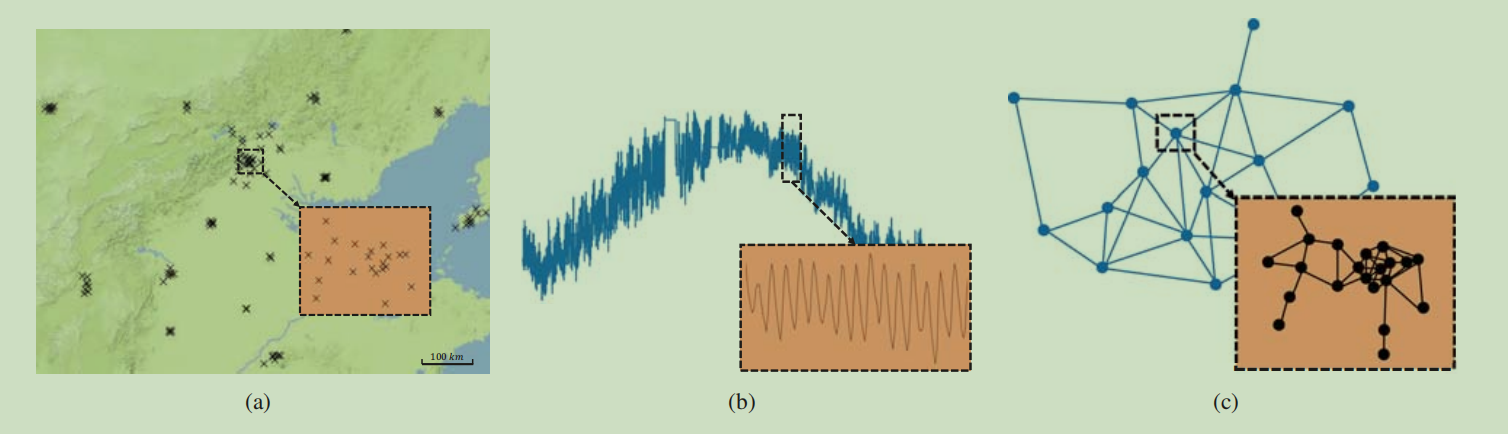如上图（b），某个传感器的读数在1年尺度上显示长期趋势，在1天尺度上显示周期性，在1小时尺度上显示波动。如上图（c）个传感器组成的结构可分为城市尺度图和区域尺度图，城市尺度图每个节点代表一个传感器，区域尺度图每个节点代表一个城市（区域>城市）。由此可见在时间和空间维度上都存在多尺度的特征，据此，作者提出了一个多尺度时空网络（MSSTN）。
网络结构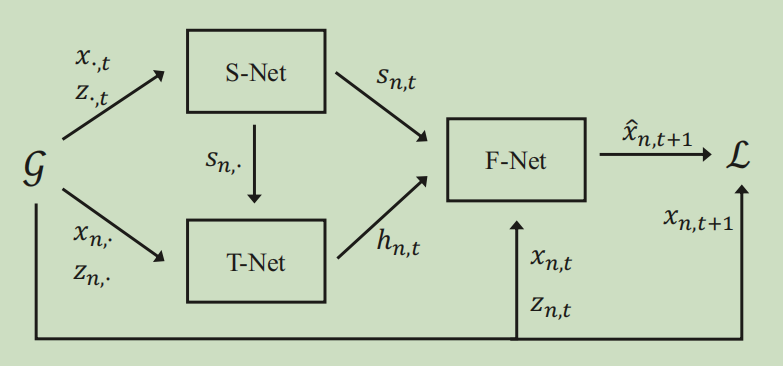MSSTN整体的网络结构比较简单，分为三个子网络：S-Net用来提取多尺度空间特征，T-Net用来提取多尺度时间特征，F-Net进行特征融合并给出最后的预测
S-Net
作者定义了多种图， 每个城市为一个图，即城市规模图，从每个城市中预先挑选一个传感器代表该城市，从而构成一个以城市为节点的区域规模图。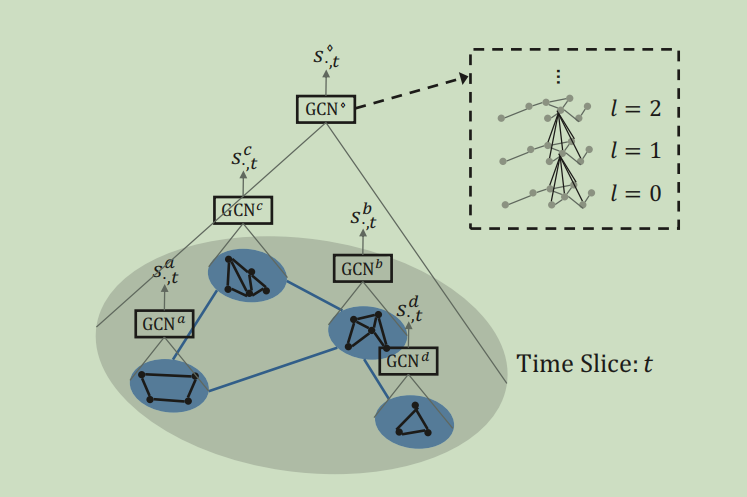对不同的图做GCN（ChebNet），得到不同图的空间特征集合。
T-Net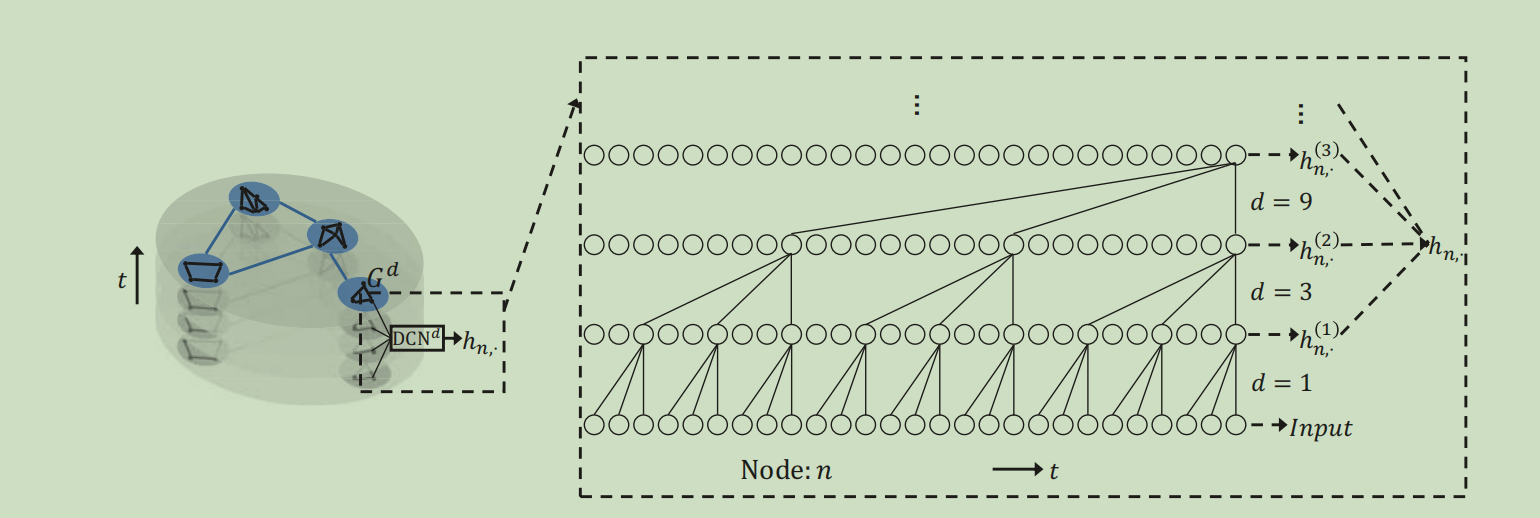T-Net的结构类似于TCN，但每层加入了一个门控非线性操作，具体公式如下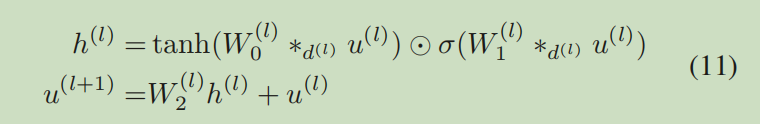u

l

u^l

为

l

l

层的输入的时序信号，

∗

d

*d

为扩张卷积，

σ

\sigma

为sigmoid激活函数
F-Net
将多尺度时间特征和空间特征和原始输入拼接后作为输入，经过一个多层感知器得到预测结果，为了确保有效的预测范围，作者采用了一个trick（

μ

\mu

律对数量化），这块不是很懂
源码
数据集分析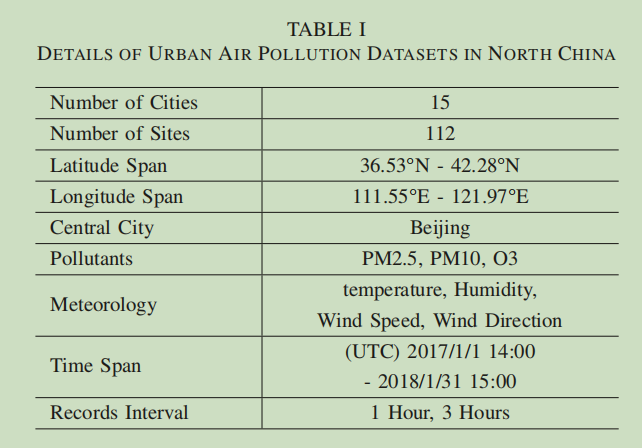数据集是以北京为中心的15个城市，文中单独提供了北京（bj）、石家庄（sjz）、太原（ty）、呼和浩特（hhht）、大连（dl）五个城市的数据，以组成城市尺度图（city-scale），加一个区域尺度（region-scale）的数据（mid、midscale）。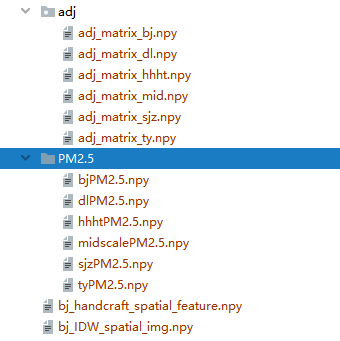PM2.5中的数据shape为（时序长度，节点数，特征数）
GeoMAN: Multi-level Attention Networks for Geo-sensory Time Series Prediction
问题提出
文章指出，基于地理的时序预测，存在两个复杂的因素：
动态时空关联性
传感器之间的相关性，即空间相关性传感器自身的相关性，即与历史时间的相关性
外部因素 传感器的读数会受到许多外部因素影响，像天气、时间等。这些因素需要考虑。
针对以上几点，作者提出了一个多级注意力网络（GeoMAN），该网络主要分为两个部分： 多级注意力机制和外部因素融合模块。 注：该网络本质还是单纯的时序预测，即每次都是对一个节点进行预测，只是预测这个结果时用到了空间的信息（所有传感器的属性值），故和交通预测直接得到多个节点的预测值有所不同。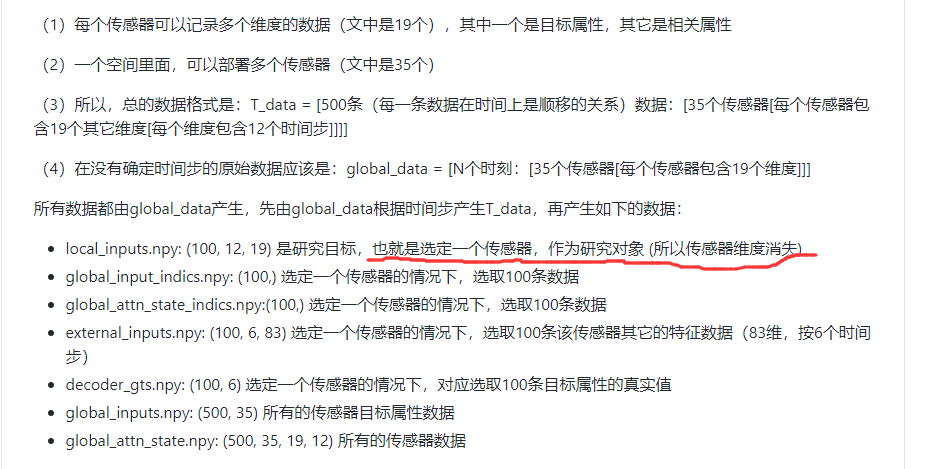网络结构
网络采用了编解码的架构，用LSTM为基础单元建模，整个网络结构图如下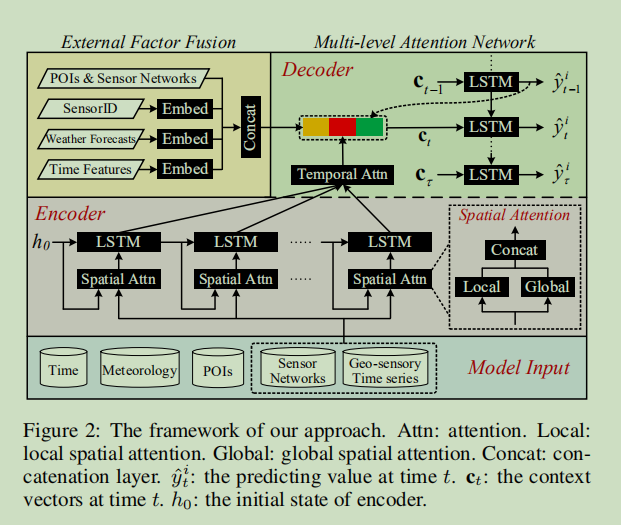空间注意力
局部空间注意力（这里的局部和图卷积上的局部不是一个概念，这里的局部就是指每个传感器多个特征，也就是争对各特征的注意力） 以下就是计算注意力的公式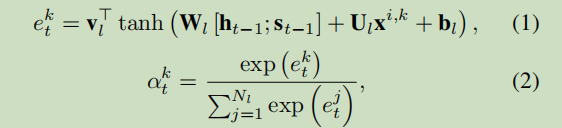h

、

s

h、s

分别是LSTM中前一时间步上的隐藏状态和细胞状态，

k

k

为第k个特征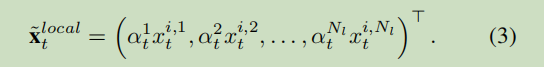这里直接将得到各注意力分数乘到各特征上。  全局空间注意力 这里的全局注意力机制就类似图注意力了（GAT），计算各节点（传感器）之间的相关性。 类似于局部空间注意力，公式如下：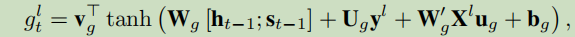l

l

为第

l

l

个传感器。 同时作者考虑到各传感器之间的地理空间相似性

P

i

,

j

P_{i,j}

（例如以距离的倒数来衡量），同时通过一个可调的超参数

λ

\lambda

来调节两个之间的作用程度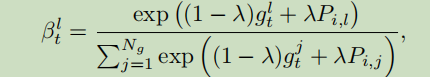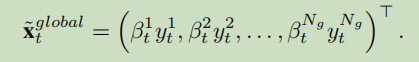编码器
编码器由多个LSTM单元组成，在输入每个LSTM单元之前都经过一个空间注意力，空间注意力将局部空间注意力和全局空间进行简单拼接。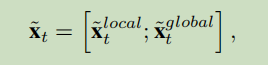时间注意力
随着编码器的长度增加，编解码的架构的性能会极速下降，为了解决这个问题，作者使用一个时间注意力机制。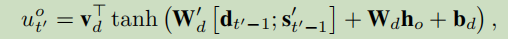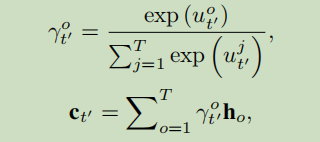外部因素融合
作者将多种外部因素（气象特征、时间特征、POIs等）引入进来，用一个简单高效的组件来融合这些因素，得到向量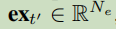解码器
解码器也是用LSTM来实现的，LSTM单元的两个输入分别是上一时间步的隐藏状态，上一步的输出、外部因素融合的向量、时间注意力的输出三者的拼接。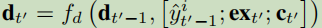最终的预测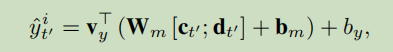展开全文数据挖掘 深度学习
• 随着我国环境监测技术的不断发展, 环境空气质量的网格化监测体系越来越受到相关工作人员的青睐, 为应对空气污染的网格化监测体系中的小型、微型监测站的空气质量预测问题, 本文提出了一种基于GCN和LSTM的空气质量...
• 基于位置数据的空气质量预测混合模型研究，对空气质量预测分类进行综述
• 针对目前空气质量预报多采用传统的数值模型现状，例如空气污染指数法，本次研究通过决策树算法以及大规模的训练数据集建立空气质量预测模型。传统的评估模型是在各种污染参数的污染分指数都计算出以后，取最大者为该...
• 从基于大数据的智能决策角度研究智能空气指数预测，引入流行的分类算法，挖掘历史数据隐含的信息，实现空气质量预测；构建了基于物联网的空气质量监测系统，利用分类算法实现实时采集数据的智能处理。针对空气指数...Android
• ## LSTM实战:空气质量预测

千次阅读 热门讨论 2020-10-17 08:33:12
LSTM实战：空气质量预测。pytorch代码，超详细。
数据集请戳： 缦旋律的资源合集

文章目录
1.原始数据预览2.数据预处理3.设置超参数4.搭建网络5.自定义dataset6.训练模型，并用val_set选出最佳模型

1.原始数据预览
原始数据是北京的空气质量数据，如下图所示：其中第二列有缺失值，但是该列是由根据其他列（具体是哪一列我忘了）的数值大小来赋值的，因此第二列可以直接删掉。
import pandas as pd
import torch
import numpy as np
from torch import optim
from torch import nn
import pickle

2.数据预处理
这个数据集比较干净，只做了归一化处理。
#原始数据预处理

data = ori.drop(columns=2)

col_names = ['date','aqi','pm2','pm10','so2','co','no2','o3']

data.columns = ['date','aqi','pm2','pm10','so2','co','no2','o3']

pro = data.iloc[:,1:]

for name in col_names[1:]:
pro[name] = (pro[name]-pro[name].min())/(pro[name].max()-pro[name].min())#归一化处理

pro['date'] = data['date']

pro.to_excel('pro_data.xlsx',index=False)


经过预处理后的数据长这样：3.设置超参数
#设置超参数
x_timesteps = 5 #用多少期去预测（在RNN模型中x_timesteps就是cell的个数）
y_timesteps = 1 #老师说一般都只预测一期，所以y_timestpes应该就是固定值1，但是后面Env我懒得改了，所以这里还是保留了y_timesteps这个超参数
stride = 2 #每次移动多少期来采样
hidden_size = 20
hidden_layers = 1
y_features = 7 #最终你想要预测多少个特征，比如我用前5期的7个特征预测滞后期的7个特征，那么y_features就是7；如果只想预测其中某个特征，那么y_features就是1
if y_features<7:
the_col_wanted =  [int(x) for x in input('输入您想要的列，若超过1列，请用,隔开（英文逗号）。您选择的列是：').split(',')] #你想要预测的特征，这个长度必须与y_features一致
if len(the_col_wanted) == y_features:
print('您最终选择的列是：',the_col_wanted)
else:
print('您的选择有误,请重新进行选择')
else:
the_col_wanted = list(range(7))

batch_size = 32
epochs = 200

4.搭建网络
#搭建网络
class Net(nn.Module):
def __init__(self):
super(Net,self).__init__()
self.lstm = nn.LSTM(input_size=7,hidden_size=hidden_size,num_layers=hidden_layers,batch_first=True)
#注意这里指定了batch_first为true哈
#这里设置了两个线性层，其实设置一层也可以
self.linear1 = nn.Linear(in_features=hidden_size,out_features=int(hidden_size/2))
self.linear2 = nn.Linear(in_features=int(hidden_size/2),out_features=y_features)
def forward(self,x,h0):
out,(h,c) = self.lstm(x)
# x的size是batch_size*x_timesteps*x_features   本例中的x_features是7
# LSTM的最终的输出是3个，h和c都是最后一个时刻的h、c
# out的size是batch_size*x_timesteps*hidden_size
# h和c 的size是(num_directions*num_layers,batch_size,hidden_size)。注意，不管有没有设置batch_first=True,batch_size永远在h和c的size的第二个。（而设置了batch_first=True之后，batch_size在output的size的第一个）
out = out[:,-1,:]  #只要最后一个cell的输出结果，out的size变为batch_size*1*hidden_size
out = out.reshape(batch_size,-1)
out = self.linear1(out)
out = self.linear2(out).reshape(batch_size,1,y_features)
#经过整个网络之后，size由batch_size*x_timesteps*x_features变成了batch_size*1*y_features
return out


5.自定义dataset
#创建数据集
class Env(Dataset):
def __init__(self,root,x_timesteps,y_timesteps,stride,mode):
super(Env,self).__init__()
self.x_timesteps = x_timesteps
self.y_timesteps = y_timesteps
self.stride = stride
self.mode = mode
self.samples = self.creat_xy('./final_sample.pkl')

self.x = self.samples[:,:-self.y_timesteps,:]
if self.y_timesteps==1:
self.y = self.samples[:,-1,the_col_wanted].reshape(len(self.x),1,y_features)
else:
self.y = self.samples[:,-self.y_timesteps:,the_col_wanted]

if self.mode == 'train':
self.x = self.x[:int(0.6*len(self.x)),:,:]
self.y = self.y[:int(0.6*len(self.y)),:,:]

if self.mode == 'val':
self.x = self.x[int(0.6*len(self.x)):int(0.8*len(self.x)),:,:]
self.y = self.y[int(0.6*len(self.y)):int(0.8*len(self.y)),:,:]

if self.mode == 'test':
self.x = self.x[int(0.8*len(self.x)):,:,:]
self.y = self.y[int(0.8*len(self.y)):,:,:]

def creat_xy(self,save_path):
#此函数用于创造sample，每个样本的size是x_timesteps+y_timesteps*7
#前面的x_timesteps*7就是放入网络中的每个样本，后面的y_timestps*7就是原始的true_y
index = 0
samples = []

while (index + self.x_timesteps + self.y_timesteps) <= (len(self.data) - 1):
single_sample = self.data[index : index + self.x_timesteps+self.y_timesteps,:]
samples.append(single_sample)
#每个single_sample的size是x_timesteps+y_timesteps*7
#前面的x_timesteps*7就是放入网络中的每个样本，后面的y_timestps*7就是原始的true_y
index += self.stride
else:
final_sample = torch.from_numpy(np.array(samples))
with open(save_path, 'wb') as f:     # 将数据写入pkl文件
pickle.dump(final_sample, f)
return final_sample

def __len__(self):
return len(self.x)

def __getitem__(self,idx):
x,y = self.x[idx,:,:],self.y[idx,:,:]

return x,y

6.训练模型，并用val_set选出最佳模型
#准备好数据
train_db = Env('./pro_data.xlsx',x_timesteps,y_timesteps,stride,'train')
val_db = Env('./pro_data.xlsx',x_timesteps,y_timesteps,stride,'val')
test_db = Env('./pro_data.xlsx',x_timesteps,y_timesteps,stride,'test')

#初始化模型、定义损失函数、优化器
model = Net()
h0,c0 = torch.zeros([hidden_layers,batch_size,hidden_size]),torch.zeros([hidden_layers,batch_size,hidden_size])
loss_fn = nn.MSELoss()
best_loss = 99999  #因为希望val_loss不断减小，所以初始的val_loss设置大一点

#设置一个evaluate函数，用于评估模型的效果(这里使用loss来衡量，根据实际情况，也可以选择precision、recall、F_β score、auc等来评估)
loss_for_all_batch = []
input_x = x.float()
true_y = y.float()
pre_y = model.forward(input_x,(h0,c0))
loss = loss_fn(pre_y,true_y) #每个batch的loss
loss_for_all_batch.append(loss)

#开始训练
for it in range(epochs):
input_x = x.float()
true_y = y.float()
pre_y = model.forward(input_x,(h0,c0))
loss = loss_fn(pre_y,true_y)
loss.backward()
optimizer.step()
if (batch_index+1)%10==0:
print('epoch：',it+1,'   batch_index:',batch_index+1,'  loss:',loss.item())

#每隔两个epoch就在val上看一下效果
if (it+1)%2 == 0:
if loss_for_val<best_loss:
print('已经完 成了{}次迭代，val的loss有所下降,val_loss为：{}'.format(it+1,loss_for_val))
best_epoch = it+1
best_loss = loss_for_val
torch.save(model.state_dict(),'best_model_ckp.txt')

print('模型已训练完成，最好的epoch是{}，在验证集上的loss是{}'.format(best_epoch,best_loss))

print('已将参数设置成训练过程中的最优值，现在开始测试test_set')
print('测试集上的loss为：',loss_for_test)

epoch： 1    batch_index: 10   loss: 0.06613270193338394
epoch： 1    batch_index: 20   loss: 0.051468655467033386
epoch： 2    batch_index: 10   loss: 0.05124117061495781
epoch： 2    batch_index: 20   loss: 0.056616220623254776
已经完 成了2次迭代，val的loss有所下降,val_loss为：0.026271890848875046
epoch： 3    batch_index: 10   loss: 0.035714808851480484
epoch： 3    batch_index: 20   loss: 0.02011280320584774
epoch： 4    batch_index: 10   loss: 0.025625046342611313
epoch： 4    batch_index: 20   loss: 0.027151888236403465
已经完 成了4次迭代，val的loss有所下降,val_loss为：0.01288842223584652
epoch： 5    batch_index: 10   loss: 0.022536305710673332
... ...
epoch： 199    batch_index: 20   loss: 0.011257675476372242
epoch： 200    batch_index: 10   loss: 0.009443326853215694
epoch： 200    batch_index: 20   loss: 0.011200820095837116
模型已训练完成，最好的epoch是54，在验证集上的loss是0.006189089734107256
已将参数设置成训练过程中的最优值，现在开始测试test_set
测试集上的loss为： 0.005623153展开全文• 基于长短期记忆神经网络模型的空气质量预测.pdf
• 基于ZigBee和循环神经网络的城市空气质量预测.pdf
• 该模型创建了一个具有LSTM网络的降噪自动编码器，以提取原始监测数据的固有空气质量特征，并对监测数据进行降噪处理，以提高空气质量预测的准确性。 将DAEDN模型中的LSTM网络结构设计为双向LSTM（Bi-LSTM），以解决...
• 基于Matlab的神经网络在绵阳空气质量预测中的应用.rar
• 基于遗传算法和BP神经网络的空气质量预测模型研究.pdf
• 基于因子分析与径向基神经网络的空气质量预测研究.pdf
• 基于时空优化的多尺度卷积神经网络空气质量预测模型.pdf
• 基于深度学习的空气质量预测模型开源代码汇总 一、传统机理空气质量模型 空气质量模型是基于人类对大气物理和化学过程科学认识的基础上，运用气象学原理及数学方法，从水平和垂直方向在大尺度范围内对空气质量进行...
基于深度学习的空气质量预测模型开源代码汇总
一、传统机理空气质量模型
空气质量模型是基于人类对大气物理和化学过程科学认识的基础上，运用气象学原理及数学方法，从水平和垂直方向在大尺度范围内对空气质量进行仿真模拟，再现污染物在大气中输送、反应、清除等过程的数学工具，是分析大气污染时空演变规律、内在机理、成因来源、建立“污染减排”与“质量改善”间定量关系及推进我国环境规划和管理向定量化、精细化过渡的重要技术方法。
二、基于深度学习的空气质量预测模型
ST-CausalConvNet A Spatiotemporal causal convolutional network for predicting PM2.5 concentrations. 代码地址：链接 论文pdf下载地址：链接 模型架构：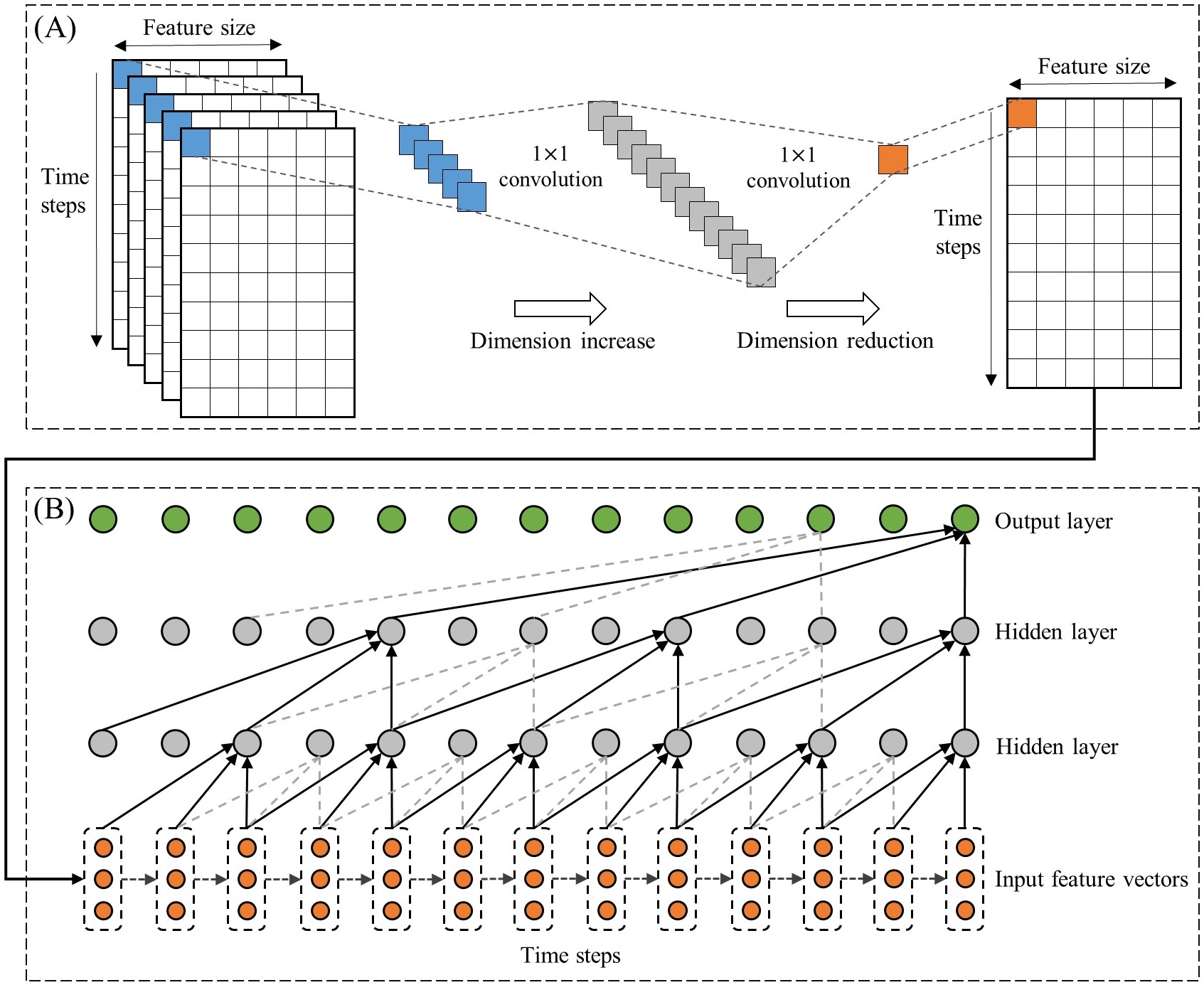GRU+CNN+ATTENTION 代码地址：链接  GRU 代码地址：链接  Seq2Seq GRU_Decode_Encode 代码地址：链接  AQI-prediction using Nested LSTM and wavelet transform (WT) AQI-prediction using Nested LSTM and wavelet transform (WT) based on Keras. 代码地址：链接  Use seq2seq and xgboost models Kdd cup data mining competition, the main task is to predict air quality(aq) in Beijing and London in the next 48 hours. Use seq2seq and xgboost models, ranking 31th in the final leaderboard. 代码地址：链接  Machine Learning techniques This project has applied some Machine Learning techniques to analyse and predict the Air Quality in Beijing. 代码地址：链接 
展开全文深度学习
• 以绵阳市2014~2016年空气污染指数(API)以及SO 2、NO 2、PM 10等污染物为研究对象,探讨了...结果表明基于BP神经网络的预报模型在污染预报中可行,并建立基于BP神经网络进行空气质量预测的预测模型,利用历史资料进行验证。
• 基于深度学习的空气质量预测方法研究，郭豪，孙岩，随着深度学习的进一步发展，该项技术也日益改善着人类的生活方式。在空气质量预测方面，可观测的数据量呈现几何倍数的大幅增长，深度学习
• 针对空气质量预测，提出了基于动态粒度小波神经网络的预测方法。为了选取合适的粒度，结合实际问题采用不断尝试的方法动态选取最优粒度，在最优粒度空间中求解问题。粒度变换后可以改变空气质量预测问题的求解空间，...
• 基于ConvLSTM的伦敦空气质量预测(1) 数据处理 实验介绍 该实验使用了ConvLSTM模型，对伦敦地区的空气质量进行了时序预测。数据集来源于开源库openair。实验的目标是预测Bloomsbury的空气污染物数值。同时，也利用了...
基于ConvLSTM的伦敦空气质量预测(1) 数据处理实验介绍
该实验使用了ConvLSTM模型，对伦敦地区的空气质量进行了时序预测。数据集来源于开源库openair。实验的目标是预测Bloomsbury的空气污染物数值。同时，也利用了Harlington, North Kensington, Marylebone 和Eltham这四个空气质量监测站的数据作为辅助预测。数据的属性有8个，分别为：NOX, NO2, NO, O3, PM2.5, 风速，风向和空气温度。
除了使用ConvLSTM, 该实验还使用了普通LSTM, BiLSTM, Attention+LSTM, LightGBM 和ARIMA进行预测。具体内容可以在github上进行查看：air_pollutants_prediction_lstm。
数据处理
读取数据。因为我的所有数据都是放在谷歌云端的。所以挂载云端读取数据
Bloomsbury=pd.read_csv('/content/drive/My Drive/air_inference/data/Bloomsbury.csv')

存放变量。用于后续的操作
sites_name=['Bloomsbury','Eltham','Harlington','Marylebone_Road','N_Kensington']
air_pollutants_list=['nox','no2','no','o3','pm2.5','ws','wd','air_temp']

sites_dic={'Bloomsbury':Bloomsbury,
'Eltham':Eltham,
'Harlington':Harlington,
'N_Kensington':N_Kensington
}

把数据展示出来。会发现存在很多空值
 def show_graph(site):
dataset=sites_dic[site]
values = dataset.values

columns = [4, 5, 6, 7, 8, 9, 10, 13]

pyplot.figure(figsize=(14,14))
i=1
for column in columns:
pyplot.subplot(len(columns), 1, i)
pyplot.plot(values[:, column])
pyplot.title(dataset.columns[column], y=0.5, loc='right')
i += 1
pyplot.show()

show_graph('Bloomsbury')

填充空值。这里主要采用了两种方法。先用一波IDW算法填充。剩余的空值直接线性插值。
#经纬度信息
coordinate_dic={'Bloomsbury':[51.52229,-0.125889],
'Eltham':[51.45258,0.070766],
'Harlington':[51.48879,-0.441614],
'N_Kensington':[51.52105,-0.213492]
}

import math
#IDW
def interpolation(lon, lat, lst,P=2):
"""
:param lon:要插值的点的x
:param lat:要插值的点的y
:param lst:lst是已有数据的数组，结构为：[[x1，y1，z1]，[x2，y2，z2]，...]
:return:返回值是插值点的值
"""
p0 = [lon, lat]
sum0 = 0
sum1 = 0
temp = []
# 遍历获取该点距离所有采样点的距离
for point in lst:
if lon == point and lat == point:
return point
Di = distance(p0, point)
# new出来一个对象，不然会改变原来lst的值
ptn = copy.deepcopy(point)
ptn.append(Di)
temp.append(ptn)

# 根据上面ptn.append（）的值由小到大排序
temp1 = sorted(temp, key=lambda point: point)
# 遍历排序的前15个点，根据公式求出sum0 and sum1
for point in temp1[0:]:
sum0 += point / math.pow(point, P)
sum1 += 1 / math.pow(point, P)

return sum0 / sum1

# 计算两点间的距离
def distance(p, pi):
dis = (p - pi) * (p - pi) + (p - pi) * (p - pi)
m_result = math.sqrt(dis)
return m_result

#填充函数
def fullfill_missing(site):
ls_temp=copy.deepcopy(sites_name)
ls_temp.remove(site)

for i in sites_dic[site].index.tolist():
for j in air_pollutants_list:
if(np.isnan(sites_dic[site].iloc[i][j])):
ls_temp2=[]
for m in ls_temp:
if(not np.isnan(sites_dic[m].iloc[i][j])):
temp_value=copy.deepcopy(coordinate_dic[m])
temp_value.append(sites_dic[m].iloc[i][j])
ls_temp2.append(temp_value)
if(len(ls_temp2)!=0):#防止所有监测站都为空
copy_dic[site].loc[i,j]=interpolation(coordinate_dic[site],coordinate_dic[site],ls_temp2)

#开始填充
fullfill_missing('Bloomsbury')
fullfill_missing('Eltham')
fullfill_missing('Harlington')
fullfill_missing('N_Kensington')

#最后线性插值
for i in sites_name:
copy_dic[i]=copy_dic[i].interpolate(method='linear', axis=0)

最后可以看到插值完的效果。红点为插值。消除噪声 这里使用的消除噪声的算法是savgol_filter
from scipy.signal import savgol_filter

for i in sites_name:
for j in air_pollutants_list:
data=copy_dic[i].loc[:,j].values
copy_dic[i].loc[:,j] = savgol_filter(data, 121, 3, mode= 'nearest')

消除完的效果如下所示数据分析
相关性检验
import numpy as np
import pandas as pd
import matplotlib.pyplot as plt
from matplotlib import gridspec
from statsmodels.graphics.tsaplots import plot_acf, plot_pacf
import pandas.util.testing as tm

dataset=dataset[['nox','no2','no','o3','pm2.5','ws','wd','air_temp']].values

def ts_plot(data):
fig = plt.figure(figsize=(14, 10))
gs = gridspec.GridSpec(2,2)

# data = np.random.normal(size=1000)

ax_ts.plot(range(len(data)), data)
plot_acf(data, ax=ax_acf, lags=30)
plot_pacf(data, ax=ax_pacf, lags=30)

plt.show()

图片总是上传失败啊。。。
数据相关性 可以看出Marlybone Road和其它地区的相关性很差哈。推测的原因是因为该地北部有一个大的park，影响了空气的流动。展开全文人工智能 机器学习 深度学习 lstm pytorch
• 针对问题一，根据提供网址查阅了西安市空气监测数据中关于空气质量优良天数、重度及以上（包括重度污染和严重污染）污染天数的统计数据并作出相应图形，基于灰色预测的方法建立了预测空气质量的模型，预测2020年西安...机器学习 人工智能 算法
• 行业分类-物理装置-基于卷积全连接的双向门控循环单元的空气质量预测方法.zip
• 以日照市为例,将 AERMOD模型应用于区域环境空气质量预测中,借助于 AERMOD多源模型分析了不同污染 源对大气环境的影响,并对未来五年日照市环境空气质量进行了预测。应用研究表明,AERMOD模型对于模拟中小城市区域......